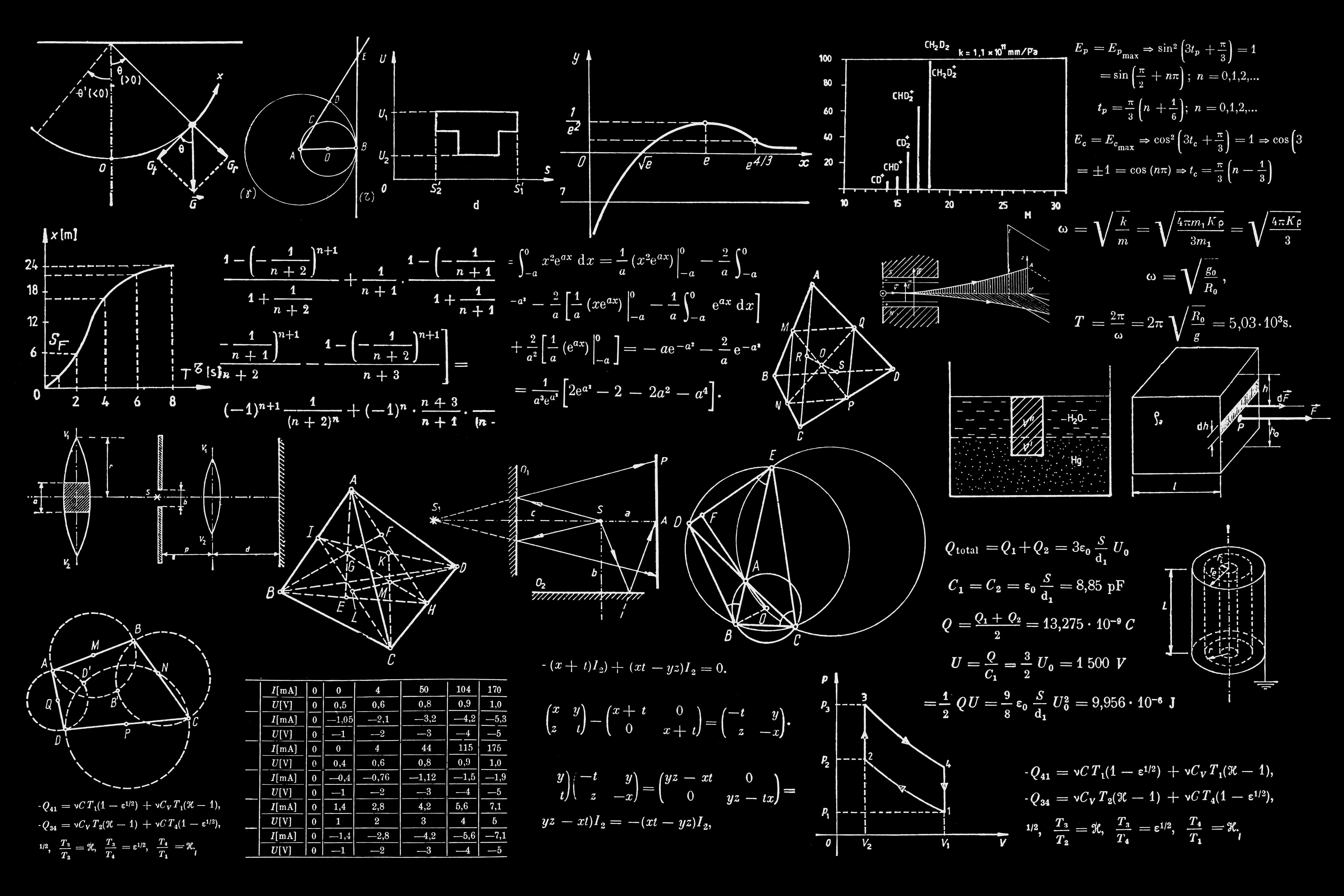# Using KaTex with AngularJS

##### by Luca Sbardella on November 06, 2014

How to use KaTex, the JavaScript library for TeX math rendering from Kahn Academy, with AngularJS

I've recently switched from using MathJax to using KaTeX when rendering maths expressions in the browser. KaTeX is a JavaScript library developed at Khan Academy where there is a lots of maths on some of their pages. The main difference between the two libraries is that, while MathJax runs asynchronously when rendering a page, KaTex blocks the browser until it has finished with the conversion. However, KaTex is much faster than MathJax during conversion which means blocking is not an issue with the added benefit that you don't see equations popping into existence while the page is processed.

I created an AngularJS directive to use in my pages:

angular.module('katex-module')
.value('mathDefaults', {
center: True,
fallback: True
})
.directive('katex', ['mathDefaults', function (mathDefaults) {

function render(katex, text, element) {
try {
katex.render(text, element);
}
catch(err) {
// MathJax fallback
if (mathDefaults.fallback)
require(['mathjax'], function (mathjax) {
if (text.substring(0, 15) === '\\displaystyle {')
text = text.substring(15, text.length-1);
element.append(text);
mathjax.Hub.Queue(["Typeset", mathjax.Hub, element]);
});
} else
}
}

return {
restrict: 'AE',

var text = element.html();
if (element.tagName === 'DIV') {
if (mathDefaults.center)
text = '\\displaystyle {' + text + '}';
}
if (typeof(katex) === 'undefined')
require(['katex'], function (katex) {
render(katex, text, element);
});
else
render(katex, text, element);
}
};
}]);


I can use the directive for inline expressions such as d y_t=\alpha_t dt+\sigma_t d W_t by inserting the mark-up:

<katex>d y_t = \alpha_t dt + \sigma_t d W_t</katex>


To create an expression in a new line one uses the div element:

<div katex>
d y_t = \alpha_t dt + \sigma_t d W_t
</div>


which renders as:

d y_t = \alpha_t dt + \sigma_t d W_t

KaTeX is more limited in the kinds of output it supports than MathJax, sticking to inline-style rendering and a much smaller subset of TeX commands. Murray Bourne has written a comparative blog post and he’s also set up a page where you can compare the output of KaTeX with MathJax.

One can use MathJax as fallback when KaTex fails to render a block of code. The fallback is implemented in the render function above and it is triggered when rendering this equation which is the Heston stochastic volatility model for asset prices

\begin{aligned} d S &= \mu S dt + \sqrt{\nu} S d W_S\\ d \nu &= \kappa(\theta - \nu) dt + \alpha \sqrt{\nu} d W_\nu\\ {\tt E}\left[dW_S d W_\nu\right] &= \rho dt \end{aligned}

These last two equations were used to create the blog-post image:

G_{\mu\nu} = 8 \pi G \left(T_{\mu\nu} - \rho_\Lambda g_{\mu\nu}\right)t' = \frac{t}{\sqrt{1 - \frac{v^2}{c^2}}}

Do you know what they are?